The FREQ Procedure
 EXACT Statement

EXACT statistic-options </ computation-options> ;
The EXACT statement requests exact tests or confidence limits for the specified statistics. Optionally, PROC FREQ computes Monte Carlo estimates of the exact p-values. The statistic-options specify the statistics to provide exact tests or confidence limits for. The computation-options specify options for the computation of exact statistics. See the section Exact Statistics for details.

Note:PROC FREQ computes exact tests with fast and efficient algorithms that are superior to direct enumeration. Exact tests are appropriate when a data set is small, sparse, skewed, or heavily tied. For some large problems, computation of exact tests might require a considerable amount of time and memory. Consider using asymptotic tests for such problems. Alternatively, when asymptotic methods might not be sufficient for such large problems, consider using Monte Carlo estimation of exact p-values. See the section Computational Resources for more information.

### Statistic-Options

The statistic-options specify the statistics to provide exact tests or confidence limits for.

For one-way tables, exact p-values are available for the binomial proportion tests and the chi-square goodness-of-fit test. Exact confidence limits are available for the binomial proportion.

For two-way tables, exact p-values are available for the following tests: Pearson chi-square test, likelihood-ratio chi-square test, Mantel-Haenszel chi-square test, Fisher’s exact test, Jonckheere-Terpstra test, and Cochran-Armitage test for trend. Exact p-values are also available for tests of the following statistics: Pearson correlation coefficient, Spearman correlation coefficient, Kendall’s tau-, Stuart’s tau-, Somers’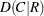, Somers’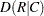, simple kappa coefficient, and weighted kappa coefficient.

For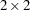tables, PROC FREQ provides exact confidence limits for the odds ratio, exact unconditional confidence limits for the proportion difference, and McNemar’s exact test. For stratifiedtables, PROC FREQ provides Zelen’s exact test for equal odds ratios, exact confidence limits for the common odds ratio, and an exact test for the common odds ratio.

Table 3.6 lists the available statistic-options and the exact statistics computed. Most of the option names are identical to the corresponding option names in the TABLES and OUTPUT statements. You can request exact computations for groups of statistics by using options that are identical to the following TABLES statement options: CHISQ, MEASURES, and AGREE. For example, when you specify the CHISQ option in the EXACT statement, PROC FREQ computes exact p-values for the Pearson chi-square, likelihood-ratio chi-square, and Mantel-Haenszel chi-square tests. You can request exact computations for an individual statistic by specifying the corresponding statistic-option from the list in Table 3.6.

Table 3.6 EXACT Statement Statistic-Options

Statistic-Option

Exact Statistics Computed

AGREE

McNemar’s test (fortables), simple kappa coefficient test,

weighted kappa coefficient test

BINOMIAL

Binomial proportion tests for one-way tables

CHISQ

Chi-square goodness-of-fit test for one-way tables;

Pearson chi-square, likelihood-ratio chi-square, and

Mantel-Haenszel chi-square tests for two-way tables

COMOR

Confidence limits for the common odds ratio and

common odds ratio test (for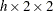tables)

EQOR | ZELEN

Zelen’s test for equal odds ratios (fortables)

FISHER

Fisher’s exact test

JT

Jonckheere-Terpstra test

KAPPA

Test for the simple kappa coefficient

KENTB

Test for Kendall’s tau-LRCHI

Likelihood-ratio chi-square test

MCNEM

McNemar’s test (fortables)

MEASURES

Tests for the Pearson correlation and Spearman correlation,

confidence limits for the odds ratio (fortables)

MHCHI

Mantel-Haenszel chi-square test

OR

Confidence limits for the odds ratio (fortables)

PCHI

Pearson chi-square test

PCORR

Test for the Pearson correlation coefficient

RISKDIFF

Confidence limits for the proportion differences (fortables)

RISKDIFF1

Confidence limits for the column 1 proportion difference

RISKDIFF2

Confidence limits for the column 2 proportion difference

SCORR

Test for the Spearman correlation coefficient

SMDCR

Test for Somers’SMDRC

Test for Somers’STUTC

Test for Stuart’s tau-TREND

Cochran-Armitage test for trend

WTKAP

Test for the weighted kappa coefficient

### Using TABLES Statement Options with the EXACT Statement

If you use only one TABLES statement, you do not need to specify the same options in both the TABLES and EXACT statements; when you specify a statistic-option in the EXACT statement, PROC FREQ automatically invokes the corresponding TABLES statement option. However, when you use multiple TABLES statements and want exact computations, you must specify options in the TABLES statements to request the desired statistics. PROC FREQ then performs exact computations for all statistics that you also specify in the EXACT statement.

The TABLES statement group option CHISQ includes tests that correspond to the following EXACT statement individual statistic-options: LRCHI, MHCHI, and PCHI. The MEASURES option in the TABLES statement includes statistics that correspond to the following EXACT statement statistic-options: KENTB, OR, PCORR, SCORR, SMDCR, SMDRC, and STUTC. The AGREE option in the TABLES statement produces analyses that correspond to the KAPPA, MCNEM, and WTKAP statistic-options in the EXACT statement. The CMH option in the TABLES statement produces analyses that correspond to the COMOR and EQOR (ZELEN) statistic-options in the EXACT statement.

### Computation-Options

The computation-options specify options for computation of exact statistics. You can specify the following computation-options in the EXACT statement after a slash (/).

ALPHA=specifies the level of the confidence limits for Monte Carlo p-value estimates. The value ofmust be between 0 and 1, and the default is 0.01. A confidence level ofproduces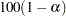% confidence limits. The default of ALPHA=.01 produces 99% confidence limits for the Monte Carlo estimates.

The ALPHA= option invokes the MC option.

MAXTIME=value

specifies the maximum clock time (in seconds) that PROC FREQ can use to compute an exact p-value. If the procedure does not complete the computation within the specified time, the computation terminates. The value of MAXTIME= must be a positive number. The MAXTIME= option is valid for Monte Carlo estimation of exact p-values, as well as for direct exact p-value computation. See the section Computational Resources for more information.

MC

requests Monte Carlo estimation of exact p-values instead of direct exact p-value computation. Monte Carlo estimation can be useful for large problems that require a considerable amount of time and memory for exact computations but for which asymptotic approximations might not be sufficient. See the section Monte Carlo Estimation for more information.

The MC option is available for all EXACT statistic-options except the BINOMIAL option and the following options that apply only toortables: COMOR, EQOR, MCNEM, RISKDIFF, and OR. PROC FREQ computes only exact tests or confidence limits for these statistics.

The ALPHA=, N=, and SEED= options also invoke the MC option.

N=n

specifies the number of samples for Monte Carlo estimation. The value of n must be a positive integer, and the default is 10,000. Larger values of n produce more precise estimates of exact p-values. Because larger values of n generate more samples, the computation time increases.

The N= option invokes the MC option.

POINT

requests exact point probabilities for the test statistics.

The POINT option is available for all the EXACT statement statistic-options except the OR and RISKDIFF options, which provide exact confidence limits. The POINT option is not available with the MC option.

SEED=number

specifies the initial seed for random number generation for Monte Carlo estimation. The value of the SEED= option must be an integer. If you do not specify the SEED= option or if the SEED= value is negative or zero, PROC FREQ uses the time of day from the computer’s clock to obtain the initial seed.

The SEED= option invokes the MC option.Previous Page | Next Page | Top of Page